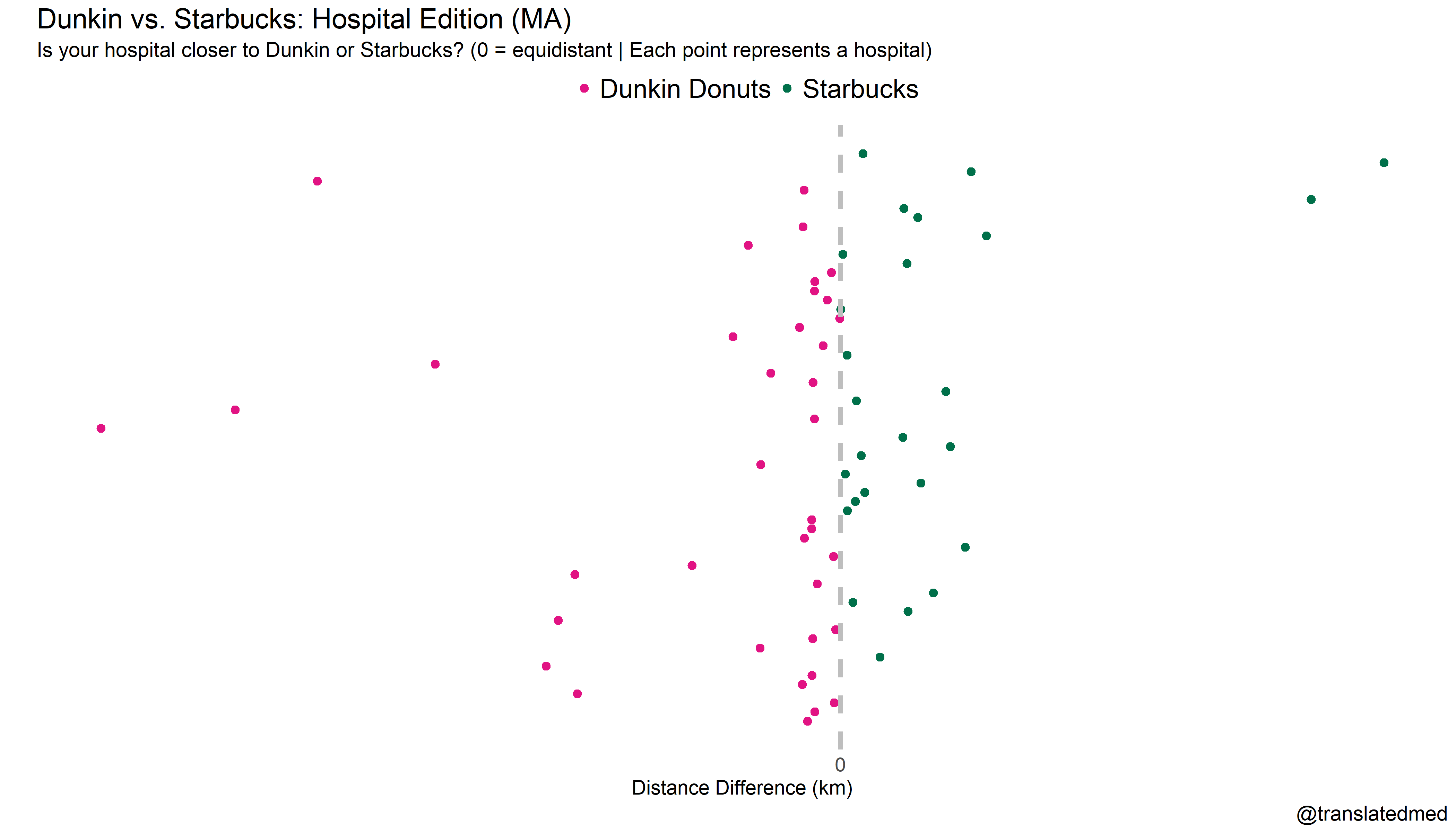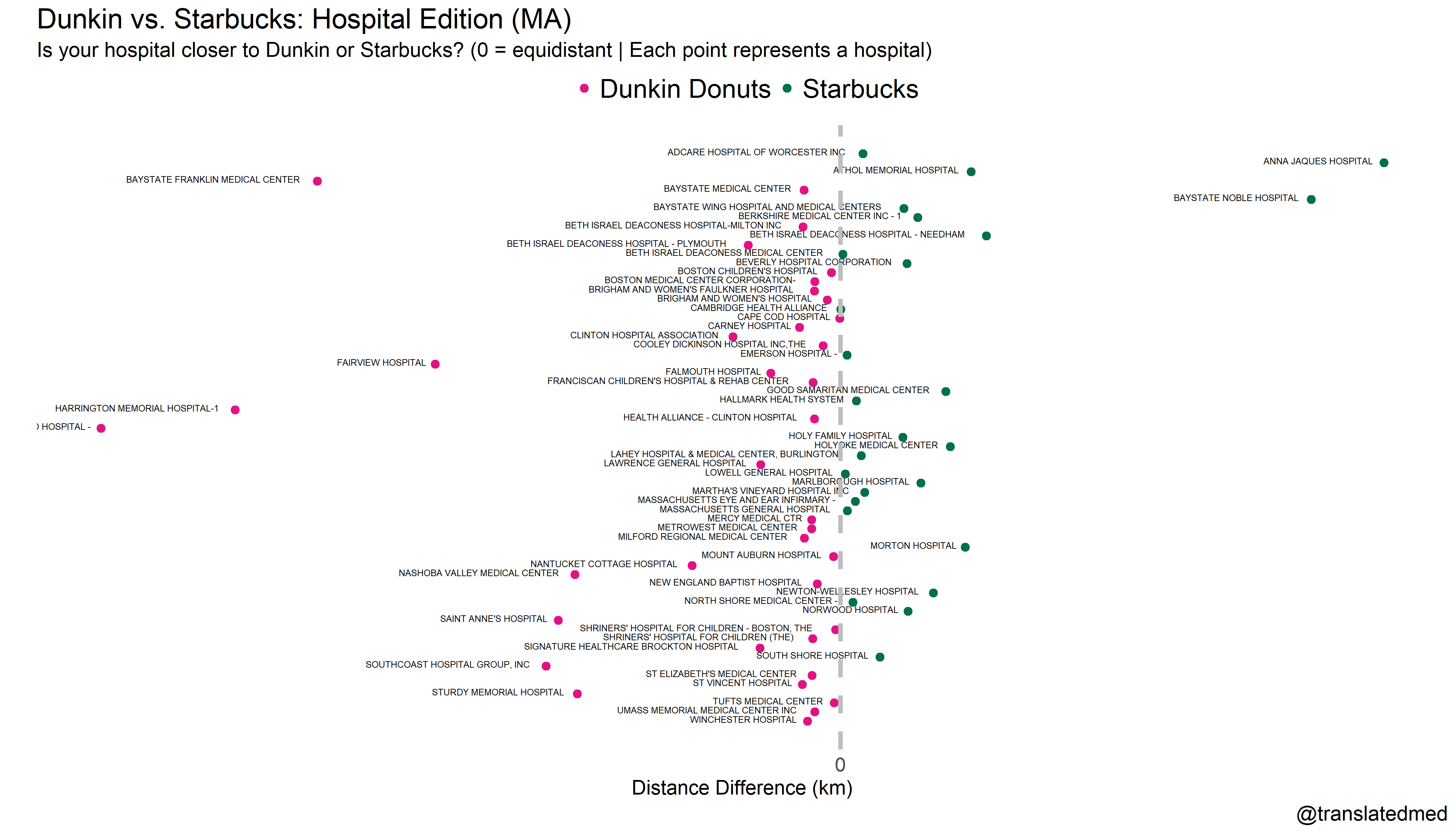# Is Your Hospital Closer to a Dunkin or Starbucks? (MA edition)

## May 13, 2018This post is inspired by #tidytuesday. Coffee is the life force for many healthcare workers. Here too, the age old question arises: Dunkin or Starbucks? Sometimes, it just comes down to proximity. We need coffee and we need it now! So, I decided to see what chain was closest to each hospital in Massachusetts.

# The Packages

``````library(tidyverse)
library(tidycensus)
library(jsonlite)
library(geosphere)``````

# The Data

``````starb = read.csv("directory.csv/directory.csv")

# The Wrangling

## Filtering for Massachusetts locations only

``````starbMA = starbUS %>% filter(State.Province == "MA")
dfHospMA = dfHosp %>% filter(State == "MA")
dunksMA = dunks %>% filter(e_state == "MA")``````

## Geocoding Hospital Location

Google provides a powerful geocoding API. More on this in a future post. The code may not be pretty, but it uses the hospital location obtain longitude and latitude.

``````dfHospMA\$lat = NaN
dfHospMA\$lon = NaN

for (i in 1:nrow(dfHospMA)) {
print(i)
dfLocation = paste0(dfHospMA\$Address[i], ",", dfHospMA\$City[i], ",", dfHospMA\$State[i])
dfLocation = gsub(' ','+',dfLocation)
dfJSON = fromJSON(dfURL)
dfHospMA\$lat[i] = dfJSON\$results\$geometry\$location\$lat
dfHospMA\$lon[i] = dfJSON\$results\$geometry\$location\$lng
}``````

Just a few corrections for geocoding errors I found.

``````dfHospMA\$lat = 42.4842322
dfHospMA\$lon  = -71.204072

dfHospMA\$lat = 41.564962
dfHospMA\$lon  = -70.621126``````

## Calcuting Distances

I am by no means an expert on calculating geospatial distances. However, from my initial reading, there are various formulas to calculate the straight line distance between 2 points. Here I use the distVincentyEllipsoid function from the geosphere package. So, I calculate the distance from each starbucks to each hospital and create a master dataframe with this data called final_df.

``````final_df = data.frame(org=NaN, dist = NaN, hospName = NaN, type = NaN)
temp_df = data.frame(org=NaN, dist = NaN, hospName = NaN, type = NaN)

for (i in 1:nrow(dfHospMA)) {
for (j in 1:nrow(starbMA)) {
x = distVincentyEllipsoid(c(starbMA\$Longitude[j], starbMA\$Latitude[j]), c(dfHospMA\$lon[i], dfHospMA\$lat[i]))
temp_df\$org = starbMA\$Store.Number[j]
temp_df\$dist = x
temp_df\$hospName = dfHospMA\$Hospital.Name[i]
temp_df\$type = "starbucks"
final_df = rbind(final_df, temp_df)
print(i)
print(x)
print("star")
}
}

for (i in 1:nrow(dfHospMA)) {
for (j in 1:nrow(dunksMA)) {
x = distVincentyEllipsoid(c(dunksMA\$loc_LONG_poly[j], dunksMA\$loc_LAT_poly[j]), c(dfHospMA\$lon[i], dfHospMA\$lat[i]))
temp_df\$org = dunksMA\$id[j]
temp_df\$dist = x
temp_df\$hospName = dfHospMA\$Hospital.Name[i]
final_df = rbind(final_df, temp_df)
temp_df\$type = "dunkin"
print(i)
print(x)
print("dunks")
}
}``````

# The Visualization

I grouped the data by hospital and chain type (Staburcks or Dunkin). Then I calculated the minimum distance to each chain and chose whether Dunkin or Starbucks was the closest. I finally calcutated the difference between the closest Starbucks and the closest Dunkin to determine, which chain was your best bet for each hospital.

Finally, I plotted the whether the hospital was closer to a Dunkin or Starbucks. As a caveat, there are 273 Starbucks in MA and 497 Dunkin in MA, so Dunkin has an advantage.

``````final_df %>% filter(!is.na(dist)) %>% group_by(hospName, type) %>% summarize(t = min(dist)) %>%
spread(type, t) %>% mutate(diff = dunkin - starbucks) %>% mutate(k =diff/1000) %>%
mutate(winner = if_else(diff < 0, "dunkin", "starbucks")) %>%
arrange(hospName) %>%
ggplot(aes(k, desc(hospName), label=hospName, color = winner)) + geom_point(size=2.5) + theme_minimal() +
scale_color_manual(values = c("#E11383", "#00704a"),
labels=c("Dunkin Donuts", "Starbucks"),
name = "") +
scale_x_continuous(labels = c("0"), breaks = c(0)) +
#geom_text(hjust=1.1, vjust=0.2, size=2.2, color="black") +
theme(axis.title.x = element_text(size = 14),
axis.text.x = element_text(size = 14),
axis.text.y = element_blank(),
title = element_text(size=16)) +
theme(panel.grid = element_blank()) +
theme(legend.position = "top",
legend.text = element_text(size=18)) +
geom_vline(xintercept=0, color="gray", linetype="dashed", size=1.5) +
labs(y="", x="Distance Difference (km)", title = "Dunkin vs. Starbucks: Hospital Edition (MA)",
subtitle = "Is your hospital closer to Dunkin or Starbucks? (0 = equidistant | Each point represents a hospital)",
caption="@translatedmed") ``````